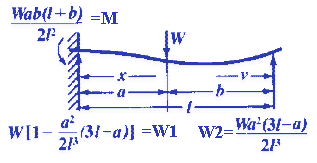Bending, Deflection and Stress Equations Calculator for
Fixed at One End, Supported at the Other, Load at any Point

Beam Deflection and Stress Formula and Calculators

Structural Beam Deflection, Stress, Bending Equations and calculator for a Beam Fixed at One End, Supported at the Other, Load at any Point.Lengths m & n
(Used in many equations)

m = (l + a)(l + b) +al
n = al(l + b)

Loads and Moment at Reaction points as Defined

Section Modulus Z

Z = I/z

I = Moment of inertia Cross Section : Units in4 (m4)
z = Distance neutral axis to extreme fiber: Units in (m)

General Stress at any Given Point

Between point of fixture and loadBetween support and loadStress at Critical Locations/PointsDeflection at any Point

Between point of fixture and loadBetween support and loadCritical DeflectionsWhere:

 E = Modulus of Elasticity psi (N/mm2) I = Moment of Inertia in4 (mm4) W = Load lbs (N) s = Stress at the cross-section being evaluated Lbs/in2 (N/mm2) y = Deflection inches (mm) x = Some distance as indicated inches (mm) u = Some distance as indicated inches (mm) a, b, c & d = Some distance as indicated inches (mm) L = Some distance as indicated inches (mm) m, n = Some calculated distance as indicated inches (mm)= Some distance as indicated inches (mm) Z = section modulus of the cross-section of the beam = I/z in3 (mm3) z = distance from neutral axis to extreme fiber (edge) inches (mm)
• Please note letter "" (lower case "L") is different than "I" (Moment of Inertia).
• Deflections apply only to constant cross sections along entire length.

References:

• Any Machinery's Handbook published since 1931 or,
• Machinery's Handbook, 21st Edition, Page 405 or,
• Machinery's Handbook, 23st Edition, Page 261 or,
• Machinery's Handbook, 27st Edition, Page 26© Copyright 2000 - 2019, by Engineers Edge, LLC www.engineersedge.comMembership Register | LoginHomeEngineering Book StoreEngineering ForumExcel App. DownloadsOnline Books & ManualsEngineering NewsEngineering VideosEngineering CalculatorsEngineering ToolboxGD&T Training Geometric Dimensioning TolerancingDFM DFA TrainingTraining Online EngineeringAdvertising CenterCopyright Notice